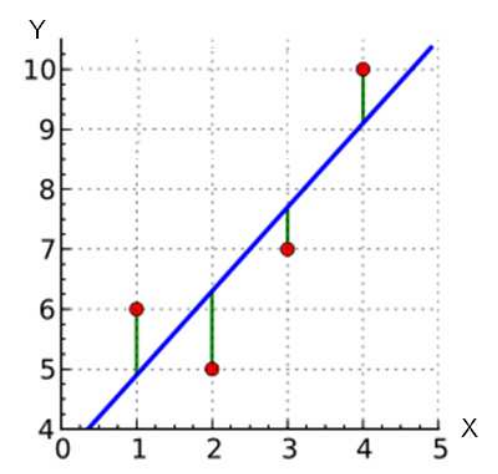시간 제한메모리 제한제출정답맞힌 사람정답 비율
1 초 128 MB71463464.151%

## 문제

Finding a line of best fit for a set of data is one of fundamental problems in statistics and numerical analysis. The problem can be formulated as follows. Suppose our data consists of a set P of n points in a plane, denoted (x1, y1), (x2, y2), (x3, y3), ..., (xn, yn). Given a line L defined by the linear equation y = ax + b, we say that the error of L with respect to P is the sum of its squared “distance” to the points in P :

$Error(L,P) = \sum_{i=1}^{n}{(y_i - ax_i - b)^2}$

The least square approach is to find the line L which minimizes such error. Your task is to write a program to find the values a and b of the line L for a given set of points P.Figure 1. Example of points and a line of best fit

## 입력

the first line contains a positive integer n (1 ≤ n ≤ 1,000). The next n lines contain 2 integers: xi and yi in each line. |xi| ≤ 106 and |yi| ≤ 106

## 출력

The output contains the values a and b in two lines respectively. The numbers must be rounded to 3 decimal places.

## 예제 입력 1

4
1 6
2 5
3 7
4 10


## 예제 출력 1

1.400
3.500